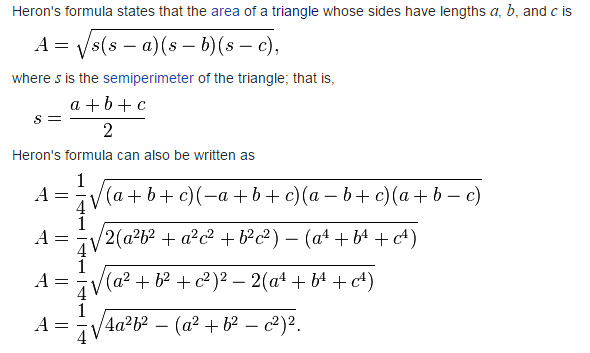# JavaScript: Find the area of a triangle where lengths of the three of its sides are 5, 6, 7

## JavaScript Basic: Exercise-4 with Solution

Write a JavaScript function to find the area of a triangle where lengths of the three of its sides are 5, 6, 7.

Sample Solution:

HTML Code:

``````<!DOCTYPE html>
<html>
<meta charset=utf-8 />
<title>The area of a triangle</title>
<body>
</body>
</html>
```
```

JavaScript Code:

``````var side1 = 5;
var side2 = 6;
var side3 = 7;
var s = (side1 + side2 + side3)/2;
var area =  Math.sqrt(s*((s-side1)*(s-side2)*(s-side3)));
console.log(area);
```
```

Sample Output:

```14.696938456699069
```

Explanation:
Calculate the area of a triangle of three given sides :
In geometry, Heron's formula named after Hero of Alexandria, gives the area of a triangle by requiring no arbitrary choice of side as base or vertex as origin, contrary to other formulas for the area of a triangle, such as half the base times the height or half the norm of a cross product of two sides.The Math.sqrt() function is used to get the square root of a number. If the value of the number is negative, Math.sqrt() returns NaN.

ES6 Version:

``````const side1 = 5;
const side2 = 6;
const side3 = 7;
const perimeter = (side1 + side2 + side3)/2;
const area =  Math.sqrt(perimeter*((perimeter-side1)*(perimeter-side2)*(perimeter-side3)));
console.log(area);
``````

Live Demo:

See the Pen JavaScript: Area of Triangle - basic-ex-4 by w3resource (@w3resource) on CodePen.

Improve this sample solution and post your code through Disqus

What is the difficulty level of this exercise?

Test your Programming skills with w3resource's quiz.

﻿

## JavaScript: Tips of the Day

Converts a string to title case

Example:

```const toTitleCase = str =>
str
.match(/[A-Z]{2,}(?=[A-Z][a-z]+[0-9]*|\b)|[A-Z]?[a-z]+[0-9]*|[A-Z]|[0-9]+/g)
.map(x => x.charAt(0).toUpperCase() + x.slice(1))
.join(' ');
console.log(toTitleCase('some_database_field_name')); // 'Some Database Field Name'
console.log(toTitleCase('Some label that needs to be title-cased')); // 'Some Label That Needs To Be Title Cased'
console.log(toTitleCase('some-package-name')); // 'Some Package Name'
console.log(toTitleCase('some-mixed_string with spaces_underscores-and-hyphens')); // 'Some Mixed String With Spaces Underscores And Hyphens'
```

Output:

```"Some Database Field Name"
"Some Label That Needs To Be Title Cased"
"Some Package Name"
"Some Mixed String With Spaces Underscores And Hyphens"
```